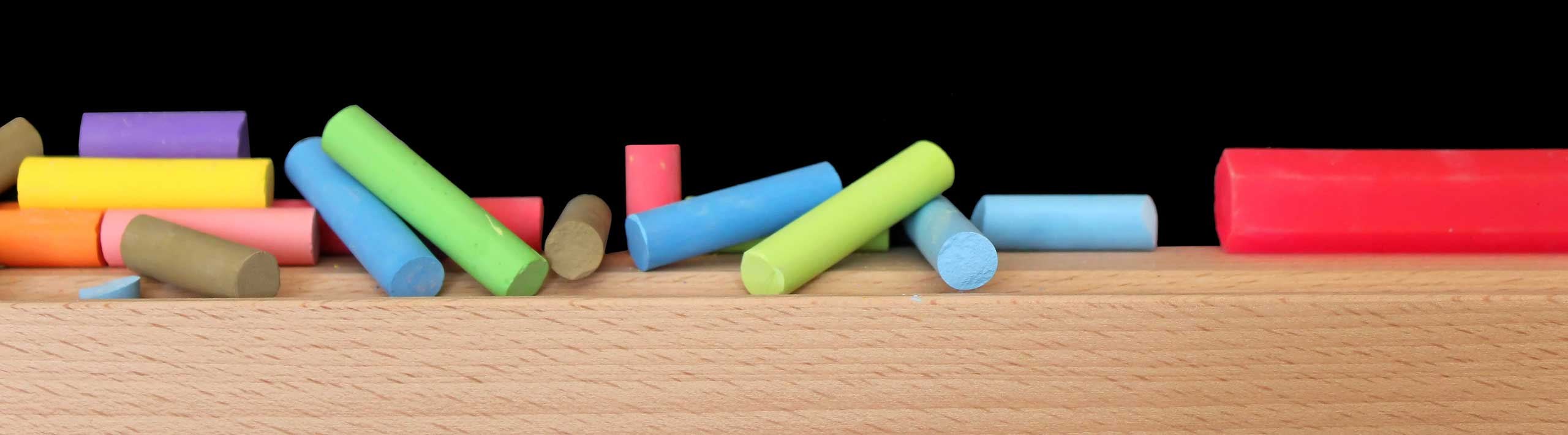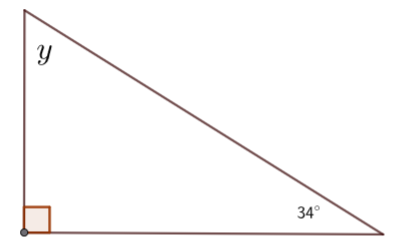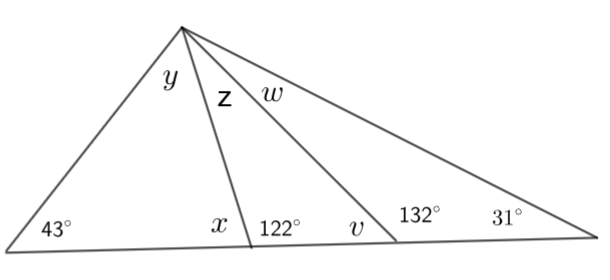# Grade 8 Questions on Angles with Solutions and Explanations

Detailed solutions and full explanations to grade 8 math questions on angles are presented.

 Find the unknown angles in the figures below.. Solution The sum of all 3 interior angles of a triangle is equal to 180�. Hence 92 + 27 + x = 180 Solve for x x = 180 - (92 + 27) = 61�. Solution The sum of all 3 interior angles of the right triangle is equal to 180�. Hence y + 34 + 90 = 180 Solve for y y = 180 - (90 + 34) = 56�. Solution Angle y and angle of measure 56� are supplementary. Hence y + 56� = 180� Solve for y y = 180 - 56 = 124� Angle x and angle of measure 144� are supplementary. Hence x + 144 = 180� Solve for x x = 180 - 144 = 36� The sum of angles x, y and z of the triangle is equal to 180� x + y + z = 180 Substitute x and y by their values found above. 36 + 124 + z = 180 Solve for z. z = 20�. Solution Let z be the third angle of the triangle on the right. The sum of the interior angles in the triangle on the right side is equal to 180�. Hence 26 + 26 + z = 180 z = 180 - 26 - 26 = 128� Angles z and y are supplementary. Hence z + y = 180 Solve for y y = 180 - z = 180 - 128 = 52� The sum of the interior angles in the angle on the left is equal to 180. Hence x + y + 64 = 180� Solve for x x = 180 - 64 - 52 = 64�. Solution Angle z and the angle of measure 133� are supplementary angles. Hence z + 133 = 180 z = 180 - 133 = 47� The angles of the lower triangle add up to 180�. Hence 33 + 133 + x = 180 x = 180 - 33 - 133 = 14� The sum of the measures of the angles of the upper triangle is equal to 180�. Hence y + z + 114 = 180 y = 180 - 114 - Z , z = 47 found before y = 180 - 114 - 47 = 19�. Solution The sum of the angles of the triangle on the right side is equal to 180�. Hence w + 131 + 32 = 180 w = 180 - 131 - 32 = 17� Angle v and the angle with measure 132� are supplementary. Hence 132 + v = 180 v = 180 - 132 = 48� The three angles of the triangle in the middle add up to 180. Hence v + z + 122 = 180 z = 180 - 122 - v z = 180 - 122 - 48 = 10� , v = 48 found above Angle x and the angle with measure 122� are supplementary. Hence x + 122 = 180 x = 180 - 122 = 58� The three angles of the triangle on the left side add up to 180. Hence x + 43 + y = 180 y = 180 - 43 - x y = 180 - 43 - 58 = 79� , x = 58 found above.